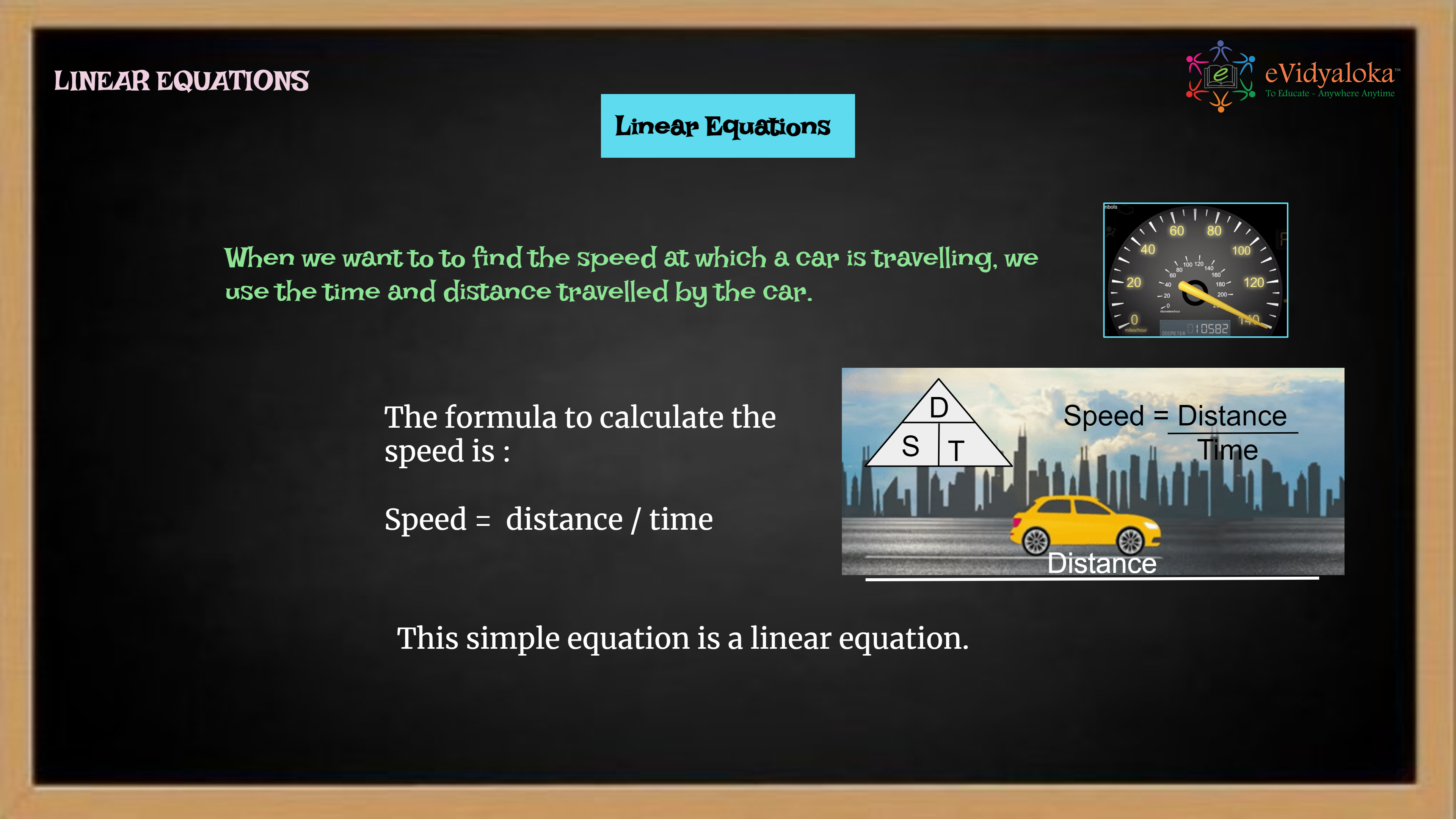# Linear Equations

``````

Dear Volunteer Teacher,

Kindly teach the bridge concept of Linear Equations prior to teaching the related textbook chapters.
Thank you.

## Course Content

• Introduction of linear equation
• Common number patterns
• Variables in arithmatic and geometry
• Algebraic expressions
• Subtraction using algebraic expression
• Multiplication using algebraic expression
• Division using algebraic expression
• Real life example of linear equations

## Learning Outcomes

Learning outcomes has been listed below which will serve as your guide for what students need to learn.

SWBAT:

• Understand Number patterns.
• Understand the use of variables in geometry and arithmatic.
• Write the expressions with variables and more than one operation in it.

## PPT Deck

To make the lesson come alive, please find below PPT deck